# Vectors

There are 366 different Starters of The Day, many to choose from. You will find below some starters on the topic of Vectors. A lesson starter does not have to be on the same topic as the main part of the lesson or the topic of the previous lesson. It is often very useful to revise or explore other concepts by using a starter based on a totally different area of Mathematics.

Main Page

### Vectors Starters:Either estimate the lengths of the red lines or, if you know how, calculate how long they are.

## Exercises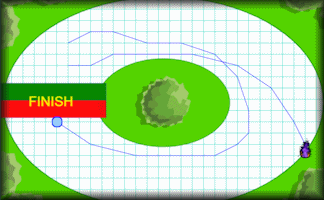#### Inertia Racetrack

A racing game for two players in which they use vectors to control the movement of their cars.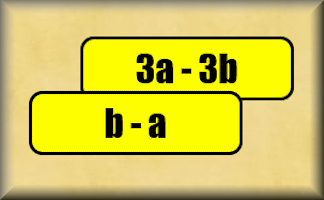#### Parallel Vectors

Collect together in groups the vectors that are parallel to each other.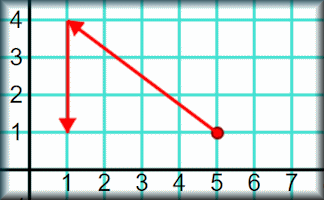#### Vector Connectors

Exercises about vectors and coordinates; using one to find the other.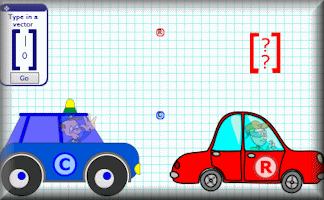#### Vector Cops

Help the cops catch the robbers by finding the vectors that will end the chase.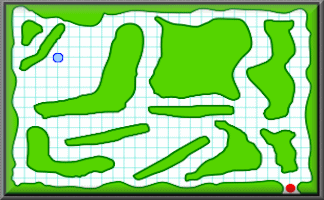#### Vector Maze

Use vectors to navigate through a maze by the shortest distance.#### Vectors

An online exercise on addition and subtraction of vectors, multiplication of vectors by a scalar, and diagrammatic representations of vectors.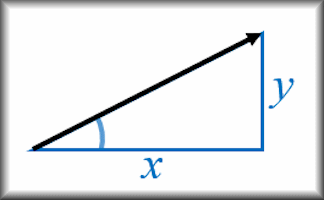#### Vectors - Magnitude and Direction

Calculate the magnitude and direction of a vector and convert between forms.

### Search

The activity you are looking for may have been classified in a different way from the way you were expecting. You can search the whole of Transum Maths by using the box below.

Have today's Starter of the Day as your default homepage. Copy the URL below then select
Tools > Internet Options (Internet Explorer) then paste the URL into the homepage field.

Set as your homepage (if you are using Internet Explorer)

Do you have any comments? It is always useful to receive feedback and helps make this free resource even more useful for those learning Mathematics anywhere in the world. Click here to enter your comments.For All: